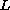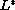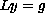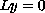Electron. J. Diff. Eqns., Vol. 2002(2002), No. 12, pp. 1-14.

### Analytic solutions of n-th order differential equations at a singular point Brian Haile

Abstract:
Necessary and sufficient conditions are be given for the existence of analytic solutions of the nonhomogeneous n-th order differential equation at a singular point. Letbe a linear differential operator with coefficients analytic at zero. Ifdenotes the operator conjugate to, then we will show that the dimension of the kernel ofis equal to the dimension of the kernel of. Certain representation theorems from functional analysis will be used to describe the space of linear functionals that contain the kernel of. These results will be used to derive a form of the Fredholm Alternative that will establish a link between the solvability ofat a singular point and the kernel of. The relationship between the roots of the indicial equation associated withand the kernel ofwill allow us to show that the kernel ofis spanned by a set of polynomials.

Submitted July 28, 2001. Published February 4, 2002.
Math Subject Classifications: 30A99, 34A30, 34M35, 46E15.
Key Words: linear differential equation, regular singular point, analytic solution.

Show me the PDF file (241K), TEX file, and other files for this article.Brian Haile Department of Mathematics and Statistics Northwest Missouri state university 800 University Drive Maryville, MO 64468 USA e-mail: bhaile@mail.nwmissouri.edu